Skinny Saturday!

Saturday, March 23
Finish a bolt of any fabric and take 25% off the price!
We are getting fabric deliveries nearly every day and running out of places to put it - we need you to take advantage of this savings to make room for more!

 table div table+table+table div table{width:100%;padding:0}table div table+table+table div table img{width:96.23%;padding:0;float:none}table div table+table+table div table td{width:100%;padding:0 1.88% 18px}/* styles */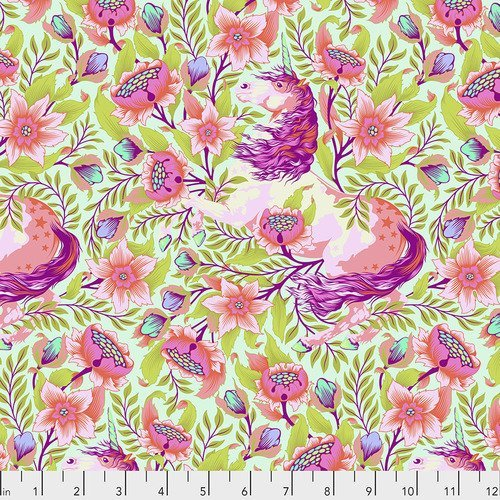Pinkerville!

Tula Pink's much anticipated fabric line, Pinkerville, has arrived!
Pinkerville is a magical place, with unicorns, sea monsters and other creatures. These fabrics are stunning, I can't wait to see what you make with them!

 table div table+table+table+table+table+table div table{width:100%;padding:0}table div table+table+table+table+table+table div table img{width:96.23%;padding:0;float:none}table div table+table+table+table+table+table div table td{width:100%;padding:0 1.88% 18px}/* styles */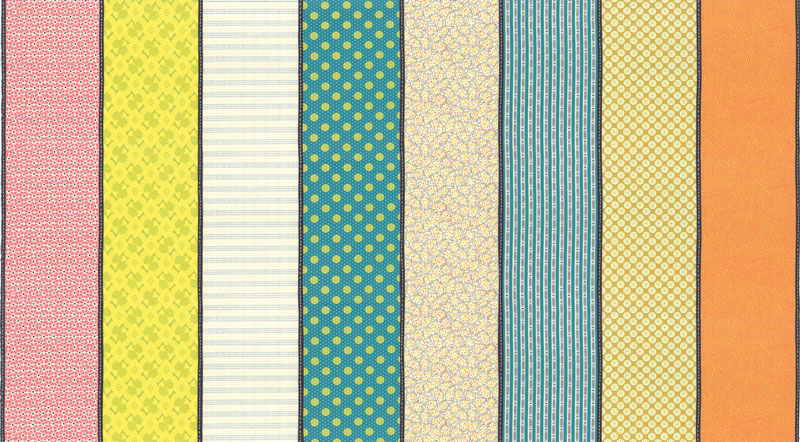Remix Lollies

Jen Kingwell is a genius for coming up with Lollies - eight different prints are on one width of fabric! Each print is 5" wide, so you can cut them into charms, or jelly roll strips, or whatever you want to make!
5 new Lollies this week and I think these are Jen's best work yet!

 table div table+table+table+table+table+table+table+table+table div table{width:100%;padding:0}table div table+table+table+table+table+table+table+table+table div table img{width:96.23%;padding:0;float:none}table div table+table+table+table+table+table+table+table+table div table td{width:100%;padding:0 1.88% 18px}/* styles */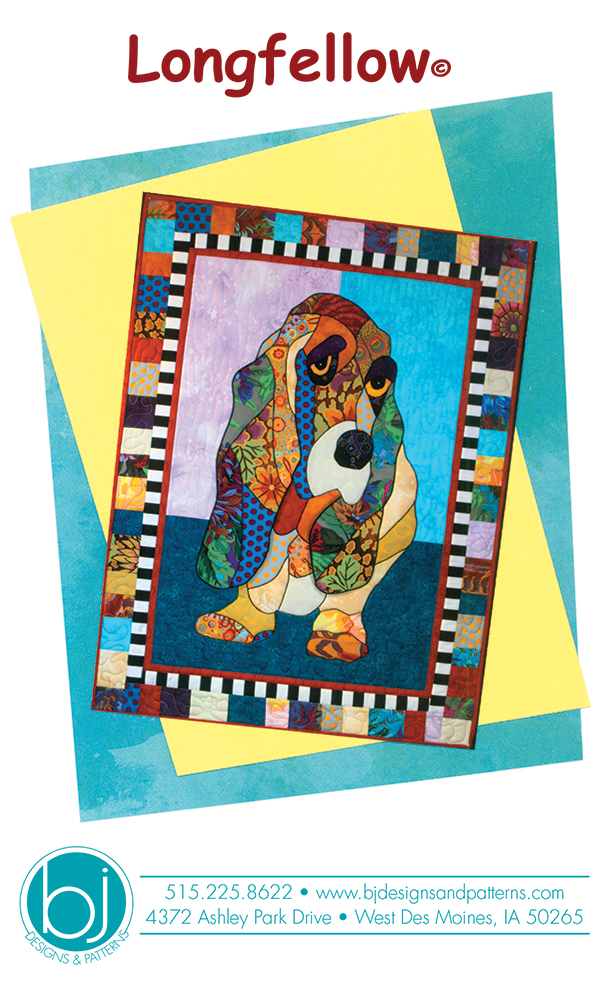New Books and Patterns!

Fuse and Fold Bags and Rugs
New Quick Curve Ruler patterns
a darling basset (had to get that one)
Summer Moon sampler - I can't wait to start on this one!
Lori Holt Vintage Christmas has super cute block designs whether you make a quilt or a smaller project
and there's a few more!

 table div table+table+table+table+table+table+table+table+table+table+table+table div table{width:100%;padding:0}table div table+table+table+table+table+table+table+table+table+table+table+table div table img{width:96.23%;padding:0;float:none}table div table+table+table+table+table+table+table+table+table+table+table+table div table td{width:100%;padding:0 1.88% 18px}/* styles */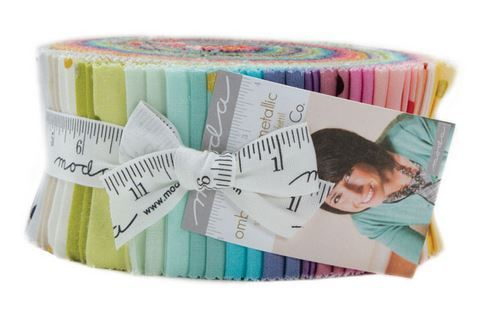New Goodies!

Ombre Metallic Jelly Rolls
Dip & Dab Roxanne's glue
Quilting Grip Gloves
Floss Flower
Quilts & Cocktails
and more!

 table div table+table+table+table+table+table+table+table+table+table+table+table+table+table+table div table{width:100%;padding:0}table div table+table+table+table+table+table+table+table+table+table+table+table+table+table+table div table img{width:96.23%;padding:0;float:none}table div table+table+table+table+table+table+table+table+table+table+table+table+table+table+table div table td{width:100%;padding:0 1.88% 18px}/* styles */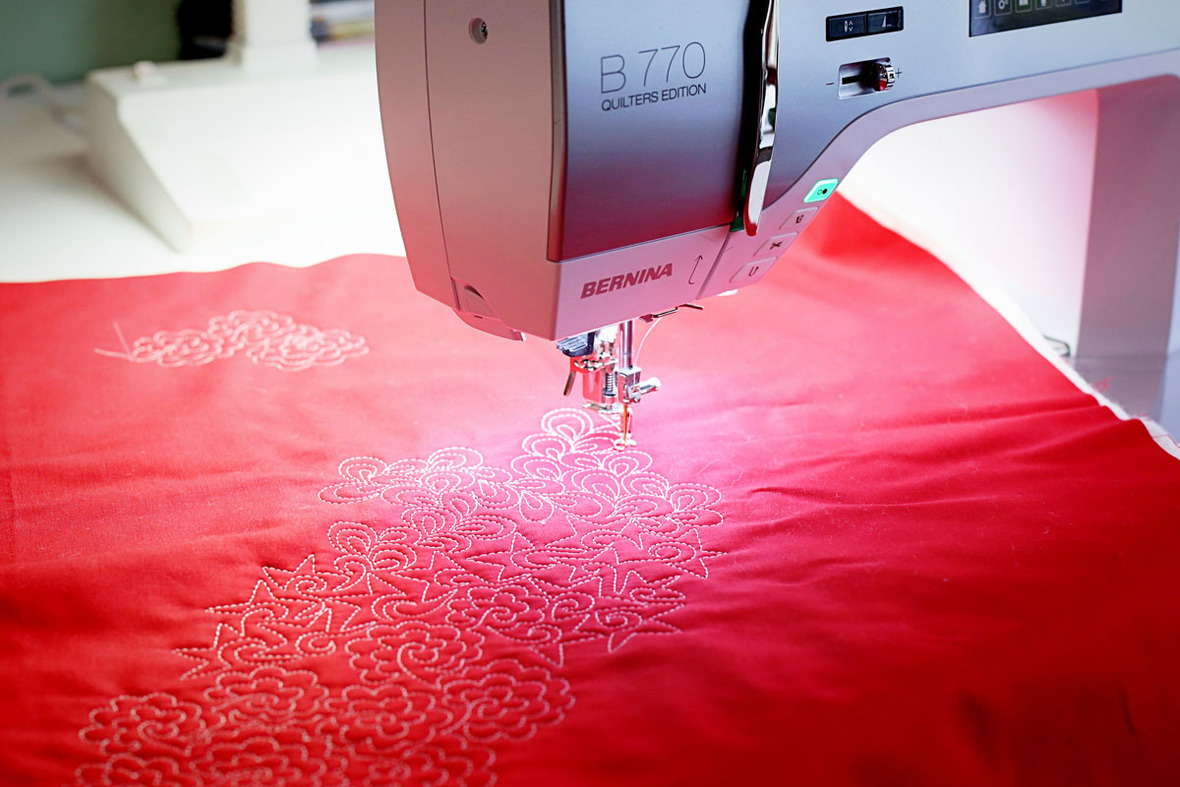Machine Quilting Teacher Needed!

We are looking for a skilled, experienced teacher for Machine Quilting classes - walking foot, free motion, ruler work - any or all.
If you are interested, please call Michelle at 941-359-3300 to make an appointment to discuss your qualifications.
We can't wait to meet you!

 table div table+table+table+table+table+table+table+table+table+table+table+table+table+table+table+table+table div table{width:100%;padding:0}table div table+table+table+table+table+table+table+table+table+table+table+table+table+table+table+table+table div table img{width:96.23%;padding:0;float:none}table div table+table+table+table+table+table+table+table+table+table+table+table+table+table+table+table+table div table td{width:100%;padding:0 1.88% 18px}/* styles */Stitch Therapy 365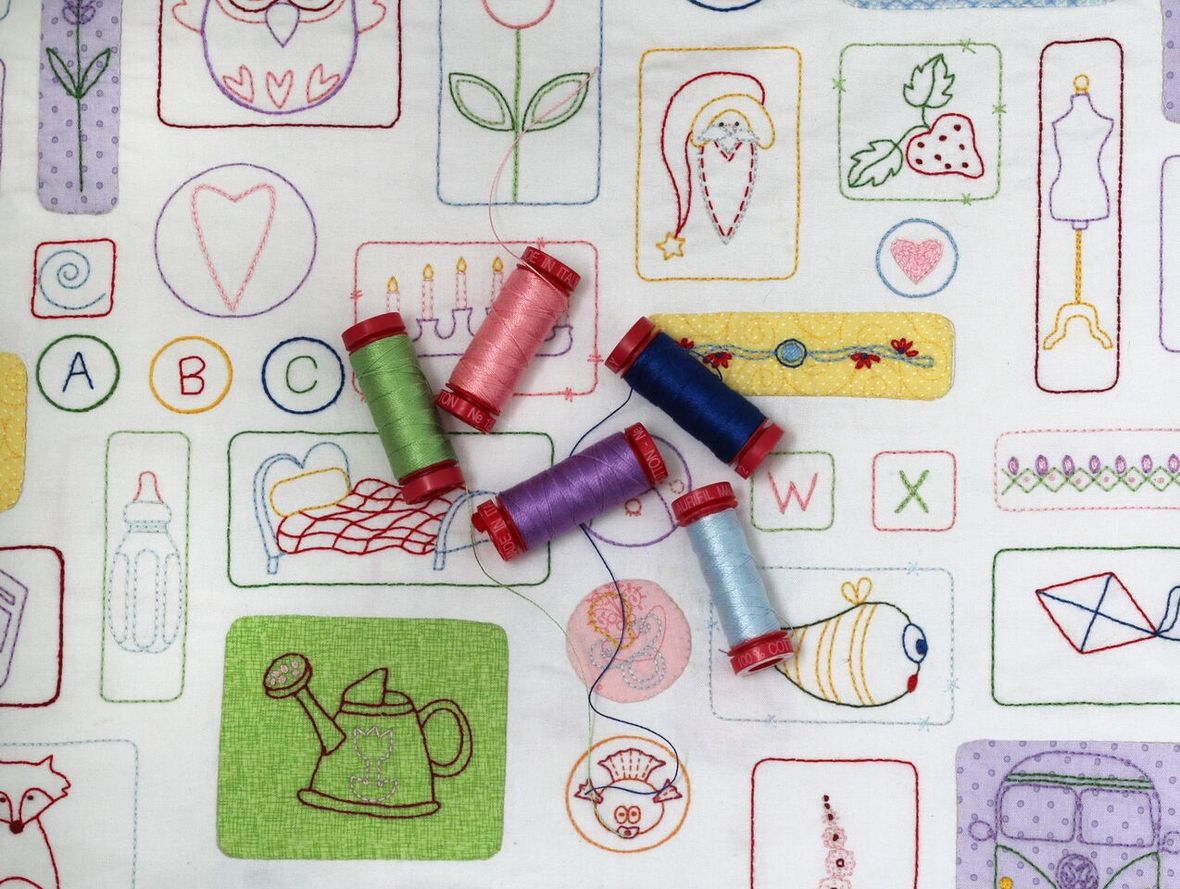It's not too late!
We have panels and thread kits AND...
your 365 days start when you get signed up, so no playing catch up, just a little stitching every day.

 table div table+table+table+table+table+table+table+table+table+table+table+table+table+table+table+table+table+table+table+table div table{width:100%;padding:0}table div table+table+table+table+table+table+table+table+table+table+table+table+table+table+table+table+table+table+table+table div table img{width:96.23%;padding:0;float:none}table div table+table+table+table+table+table+table+table+table+table+table+table+table+table+table+table+table+table+table+table div table td{width:100%;padding:0 1.88% 18px}/* styles */Next PMS Night is Friday, April 19

6pm to midnight
Treat yourself to a night of food, fabric and friends!

 table div table+table+table+table+table+table+table+table+table+table+table+table+table+table+table+table+table+table+table+table+table+table+table div table{width:100%;padding:0}table div table+table+table+table+table+table+table+table+table+table+table+table+table+table+table+table+table+table+table+table+table+table+table div table img{width:96.23%;padding:0;float:none}table div table+table+table+table+table+table+table+table+table+table+table+table+table+table+table+table+table+table+table+table+table+table+table div table td{width:100%;padding:0 1.88% 18px}/* styles */Casual Sew 10am to 4pm

Friday, March 22, 29, April 5, 12, 19, 26

Upcoming Classes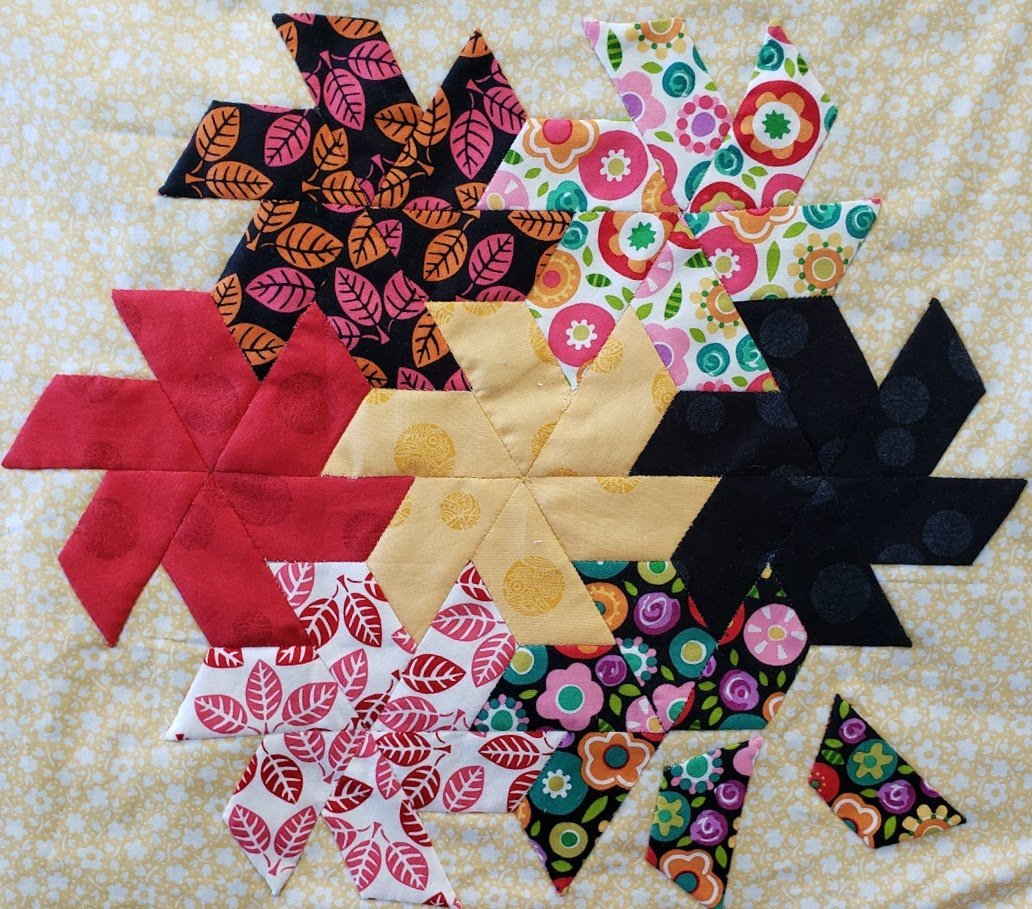EPP Gathering in the Afternoon!

Sounds like a great day for all our hand stitching lovers!
Take one or both of the classes on Wednesday, March 27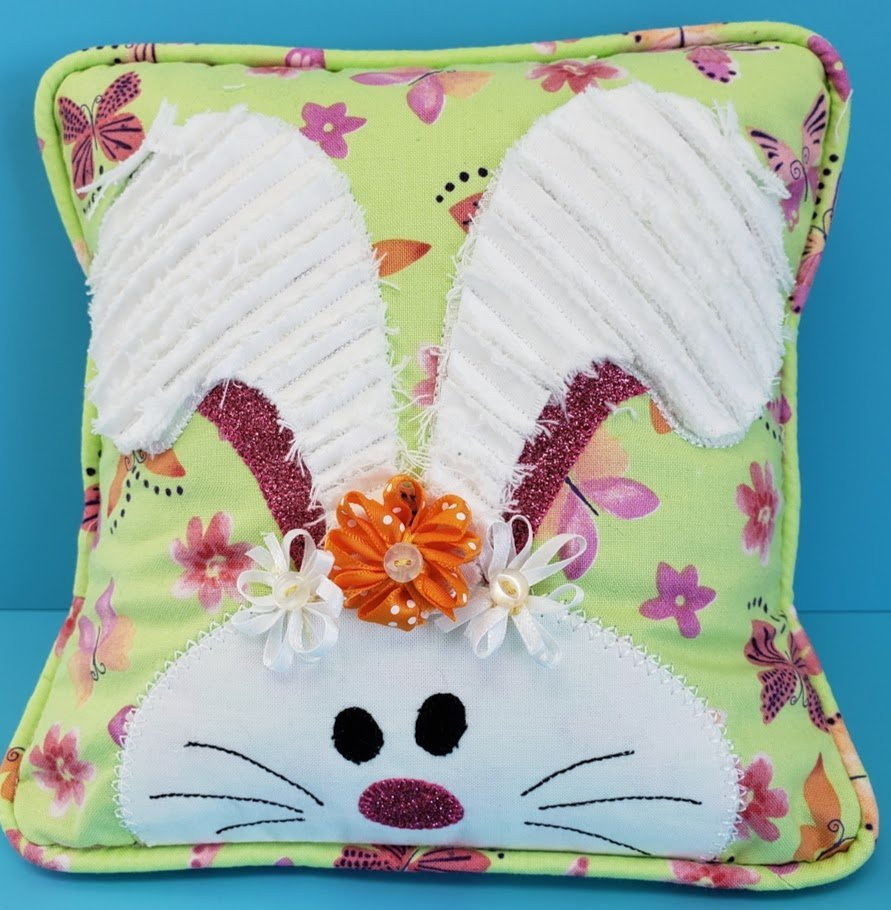Bench Buddies Bunny Pillow

Make the adorable Bench Buddy 8” pillow!.
You will learn chenille technique and make some cute ribbon flowers to adorn the pillow.
Class is Thursday, March 28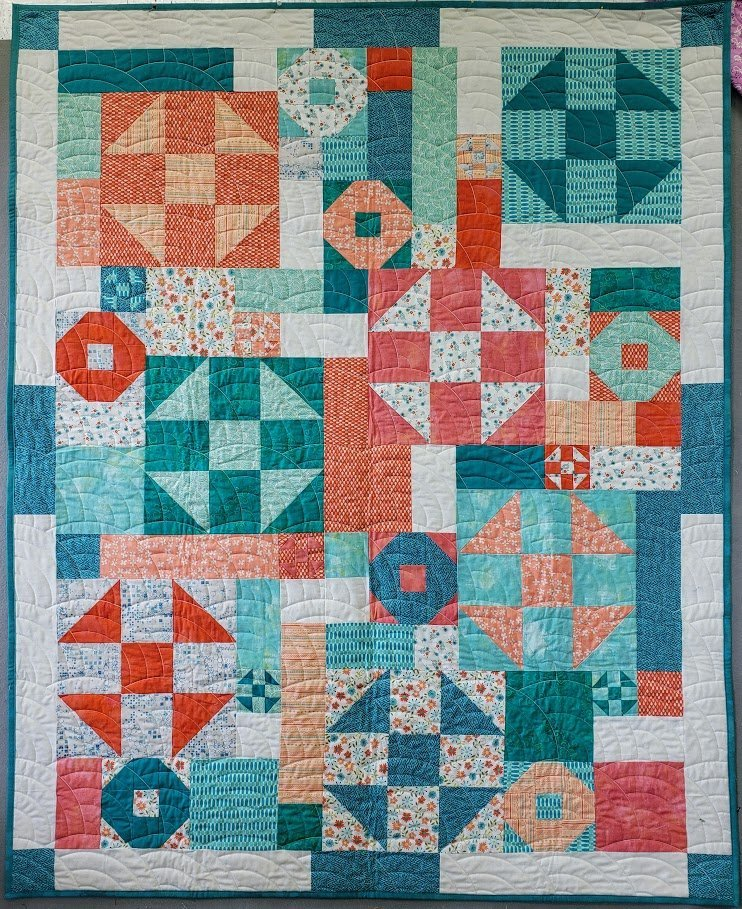Fourteen Hugs and Kisses

With squares, rectangles, and half-square triangles, you can make hugs blocks or kisses blocks or a combination of the two.
Class is Saturday, March 30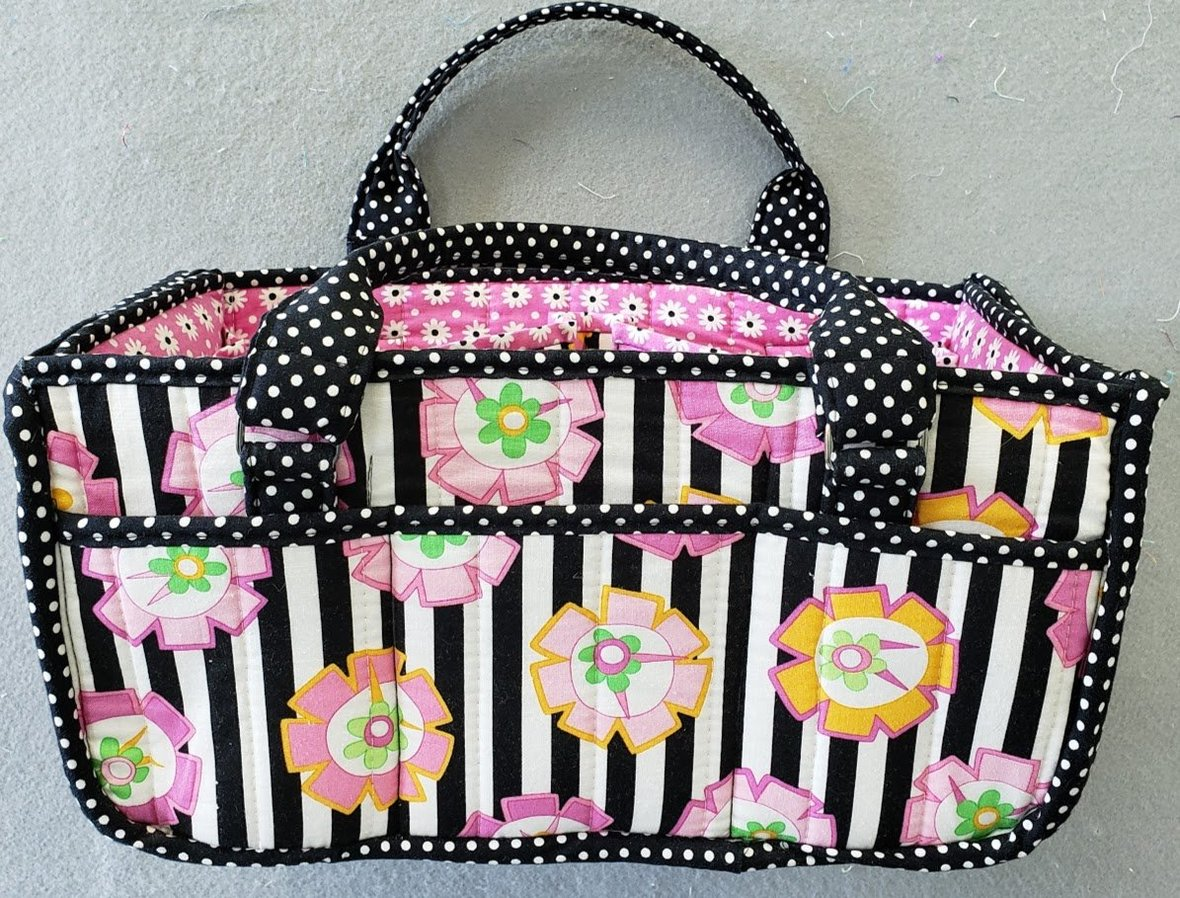This sturdy organizer has lots of pockets and bellowed inner dividers to hold all your supplies and tools.
Class is Monday, April 1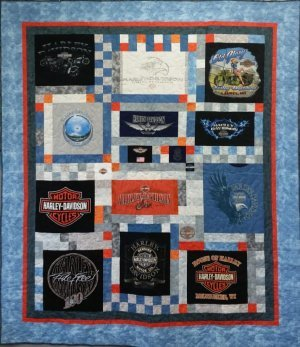T-Shirt Quilt Class

One of our most popular classes is being offered on April 3 and 10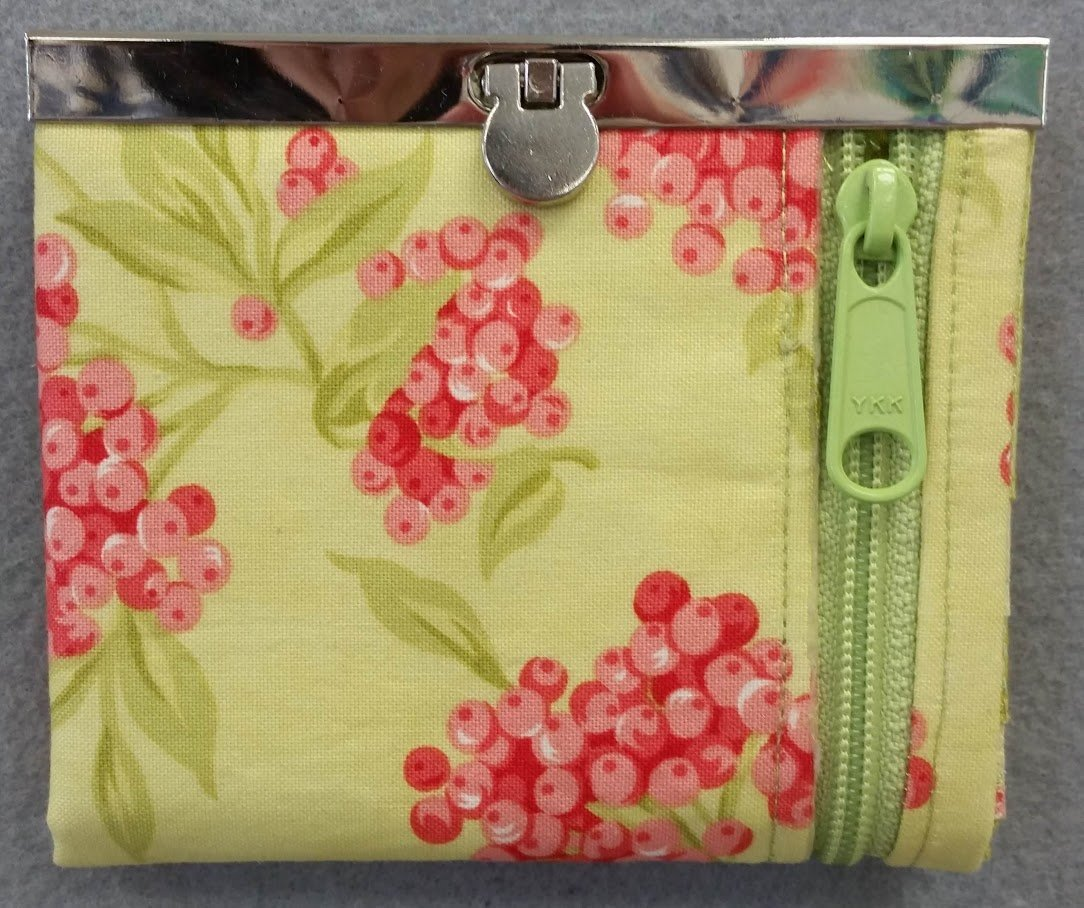Mini Diva Wallet

Adorable mini wallet with zippered pockets and a metal wallet frame
class is Thursday, April 4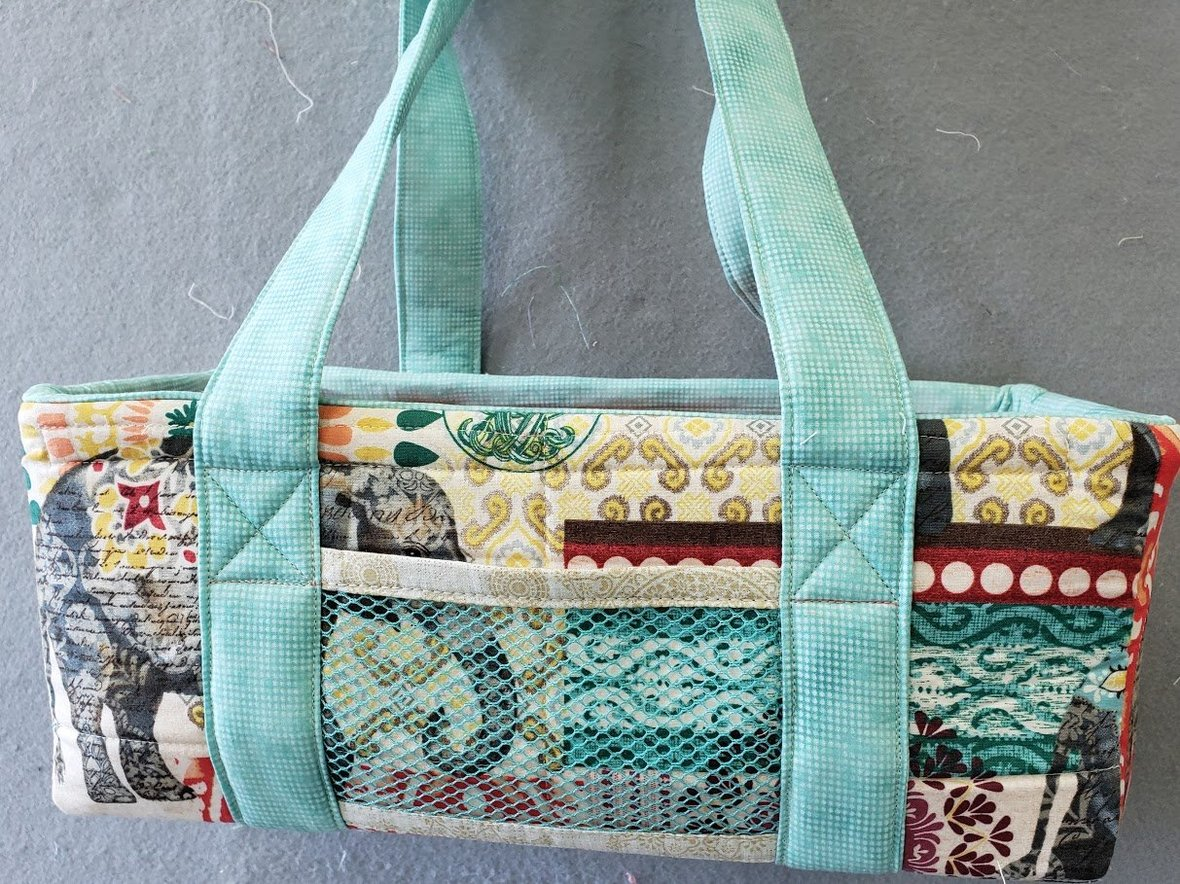Wire Framed Tote

Be organized in style with a wire framed tote - choose from 3 sizes.
class is Monday, April 8

And there's more!

 table div table+table+table+table+table+table+table+table+table+table+table+table+table+table+table+table+table+table+table+table+table+table+table+table+table+table+table+table+table+table+table+table+table+table+table+table+table div table{width:100%;padding:0}table div table+table+table+table+table+table+table+table+table+table+table+table+table+table+table+table+table+table+table+table+table+table+table+table+table+table+table+table+table+table+table+table+table+table+table+table+table div table img{width:96.23%;padding:0;float:none}table div table+table+table+table+table+table+table+table+table+table+table+table+table+table+table+table+table+table+table+table+table+table+table+table+table+table+table+table+table+table+table+table+table+table+table+table+table div table td{width:100%;padding:0 1.88% 18px}/* styles */Open 7 days a week for your convenience!

Monday through Saturday, 10am to 5pm
Sunday 12pm to 4pm

See you soon!

 table div table+table+table+table+table+table+table+table+table+table+table+table+table+table+table+table+table+table+table+table+table+table+table+table+table+table+table+table+table+table+table+table+table+table+table+table+table+table+table div table{width:100%;padding:0}table div table+table+table+table+table+table+table+table+table+table+table+table+table+table+table+table+table+table+table+table+table+table+table+table+table+table+table+table+table+table+table+table+table+table+table+table+table+table+table div table img{width:96.23%;padding:0;float:none}table div table+table+table+table+table+table+table+table+table+table+table+table+table+table+table+table+table+table+table+table+table+table+table+table+table+table+table+table+table+table+table+table+table+table+table+table+table+table+table div table td{width:100%;padding:0 1.88% 18px}/* styles *//* styles */ Maggie likes her jammies on these chilly nights!
 table.module-40{width:78.11%;padding:0}table div table+table+table+table+table+table+table+table+table+table+table+table+table+table+table+table+table+table+table+table+table+table+table+table+table+table+table+table+table+table+table+table+table+table+table+table+table+table+table+table+table div table{width:78.11%;float:none;margin-left:auto;margin-right:auto;padding:0}table div table+table+table+table+table+table+table+table+table+table+table+table+table+table+table+table+table+table+table+table+table+table+table+table+table+table+table+table+table+table+table+table+table+table+table+table+table+table+table+table+table div table a{border:0 none;text-decoration:none}table div table+table+table+table+table+table+table+table+table+table+table+table+table+table+table+table+table+table+table+table+table+table+table+table+table+table+table+table+table+table+table+table+table+table+table+table+table+table+table+table+table div table img{width:100%!important;border:0 none;text-decoration:none}table div table+table+table+table+table+table+table+table+table+table+table+table+table+table+table+table+table+table+table+table+table+table+table+table+table+table+table+table+table+table+table+table+table+table+table+table+table+table+table+table+table div table td{width:100%;padding:0}/* styles */
 Like   Pin   +1